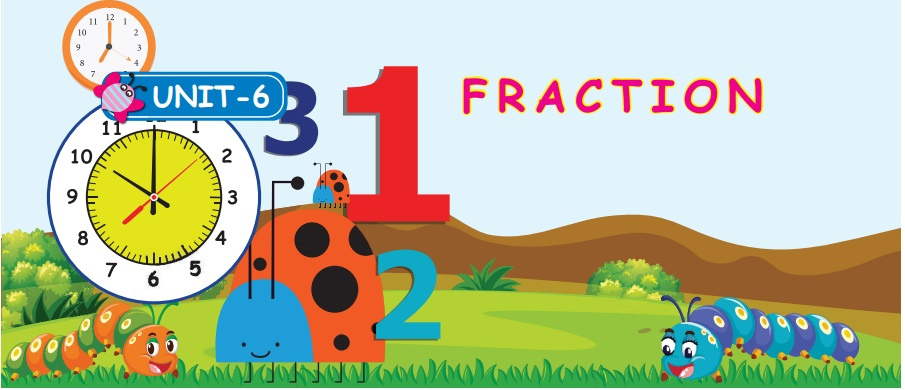Home | | Maths 4th Std | Introduction to natural fractions

# Introduction to natural fractions

Able to observe items being a part or parts of a whole.

UNIT 6

FRACTIONIntroduction to natural fractions

• Able to observe items being a part or parts of a whole.Introduction:

Raja bought a cake for his four Children. He has to make it four equal parts. Then he took a knife and slicked it into four equal parts. Then he gave it to his Children they had eaten it happily.Look at the following pictures keenlyFlag, Number rod, Chocolate, Palm fruit, Label, Leaf

Note:

The Pictures given above are divided into equal parts.

Can we fill this?The Following Shapes are divided into equal partsTags : Fraction | Term 2 Chapter 6 | 4th Maths , 4th Maths : Term 2 Unit 6 : Fraction
Study Material, Lecturing Notes, Assignment, Reference, Wiki description explanation, brief detail
4th Maths : Term 2 Unit 6 : Fraction : Introduction to natural fractions | Fraction | Term 2 Chapter 6 | 4th Maths